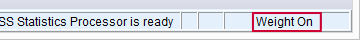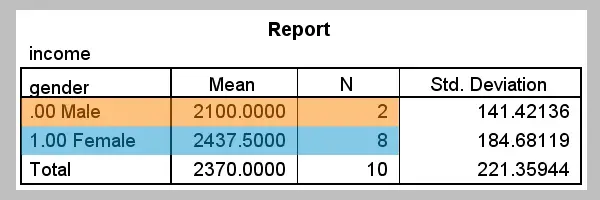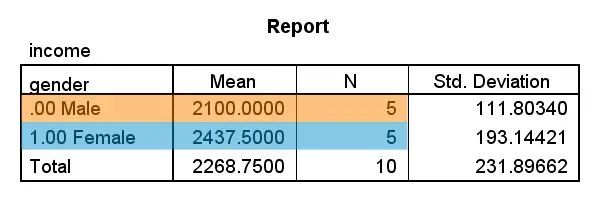SPSS TUTORIALS BASICS ANOVA REGRESSION FACTOR CORRELATION

# SPSS WEIGHT Command

By default, every case in your data counts as a single case. However, you can have each case count as more or less than one case as well. This is called weighting.
For instance, the first case in your data may count as 2 cases and the second one as .5 cases. These numbers, the case weights, are contained in a weight variable. Running `WEIGHT BY [...]` tells SPSS to treat the values of some weight variable as the active case weights. Note that the status bar informs you whether weighting is in effect or not.## SPSS Weight - Basic Use

Similarly to `SPLIT FILE` and `FILTER`, `WEIGHT` has three main commands.

1. `WEIGHT BY [...].` switches a weight variable on. If a weight variable is already in effect, it can be used for setting a different variable as the active case weights.
2. `SHOW WEIGHT.` shows which variable is currently used as the weight variable.
3. `WEIGHT OFF.` switches the case weights off. After doing so, every case counts as a single case again.

## SPSS Weight - Caveats

• In contrast to `SPLIT FILE` and `FILTER`, the active weight variable is saved with the data. So when you start SPSS and open a data file, a weight variable may already be in effect.
• An active weight variable does not only affect the output that's generated. Some data modifications are also influenced by case weights (most notably `AGGREGATE`).
• Some users inspect which weight variable is in effect from the menu. When seeing current status: Weight cases (...), they agree with that and click . However, this turns the weight variable off.Accidentally turning case weights off

## Why Would you Weight Cases?

The main scenarios in which you'll want to weight your cases are the following:

1. Your sample is not representative for the population you're investigating. For example, you may know that 50% of your target population consist of females but you have 80% females in your sample. In this case you can weight down these 80% of females to 50% of your sample by assigning case weights of .625 to them. Similarly, you can weight up the 20% male respondents to 50% of your sample as well by using weights of 2.5.
Note that these weights don't correspond to the numbers of observations actually made. In this scenario, weights typically have a mean of 1 so the weighted sample size is exactly equal to the unweighted sample size. We'll demonstrate this scenario with the example below.
2. In some cases you only have aggregated data. A typical example is a contingency table ("crosstab") presented in a book or article. In this case, case weights will al be positive integers. In this case, weights correspond to the numbers of observations that were actually made.
3. You may trick SPSS by using weights in some cases but this is beyond the scope of this tutorial.

## SPSS Weight - Example

“We held a small survey on income. Unfortunately, 80% of our respondents are female while this is 50% of our target population. That is, our sample is not representative for our population because female respondents are overrepresented.”

Running the syntax below creates these data and computes mean incomes for male, female and all respondents.

*1. Create some test data.

data list free / case_weight gender income.
begin data
2.5, 0, 2200, 2.5, 0, 2000, 0.625, 1, 2700, 0.625, 1, 2300, 0.625, 1, 2400, 0.625, 1, 2700, 0.625, 1, 2400, 0.625, 1, 2300, 0.625, 1, 2500, 0.625, 1, 2200
end data.

value labels gender 0 'Male' 1 'Female'.

*2. Unweighted mean incomes.

means income by gender.

## Biased Estimate for Unweighted CasesFemale respondents overrepresented and having higher incomes

Note in the screenshot above that female respondents have higher average incomes and are overrepresented as well. The result of this is that the estimated mean income for the entire target population (`€ 2370,-`) is biased upwards. We can correct this by weighting our respondents as described earlier. The syntax below demonstrates how to do so.

*3. Weight cases + quick check, then run weighted mean incomes.

weight by case_weight.

show weight.

means income by gender.

*4. Switch off weight and do quick check on it.

weight off.

show weight.

## Unbiased Estimate for Weighted CasesFemales and males equally represented when weight in effect

In the screenshot above, first take a look at the sample sizes. They're now equal for females and males, thus rendering the sample representative of the target population with regard to gender. Also note that the total sample size is still `10`. This is because the average case weight is exactly one. Second, the estimated mean income for our target population is now `€ 2268,75-`. This is because we correct for the aforementioned upwards bias by weighting.

# Tell us what you think!

*Required field. Your comment will show up after approval from a moderator.

# THIS TUTORIAL HAS 13 COMMENTS:

•### By Ruben Geert van den Berg on August 11th, 2017

Hi Jeteendra!

Yes, your syntax looks great! Also see IF.

•### By Aubrey Daniel on May 24th, 2018

Is it right to use weight when calculating likert type data using chi-square test?

•### By Ruben Geert van den Berg on May 24th, 2018

Hi Aubrey!

Did you mean if it's ok to run a chi-square independence test on 2 Likert items with WEIGHT on?

There's 2 basic scenarios:

-If the WEIGHT variable contains frequencies (all positive integers > 1) that really indicate the number of people who gave some answer, you can safely test for statistical significance -including chi-square tests.

-The WEIGHT variable contains non-integer values (such as 0.8, 1.2, 2.3 and so on) with an average of one. Each case in your data represents 1 person but the WEIGHT variable is used for rendering the sample (more) representative of some target population. In this case, all statistical significance tests (including chi-square) will be biased. In this case you should use the SPSS complex samples option (module) for the correct results.

Hope that helps!